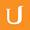## Eulers Number - Differential Equations in Action

• 0:00 - 0:08
So now that we now that the slope of two to the power of X at X equals zero is slightly less than one,
• 0:08 - 0:14
and the slope of three to the power of X at X equals zero is slightly larger than one,
• 0:14 - 0:21
so it's not hard to imagine that somewhere in between two and three, there is a number called E--
• 0:21 - 0:28
Euler's number--so that E to the power of X has a slope of exactly one at X equals zero.
• 0:28 - 0:40
This function is called The Exponential Function. Whereas two to the power of X and three to the power of X are just exponential functions.
• 0:40 - 0:46
As this exponential function, The Exponential Function is so important, it gets a special name;
• 0:46 - 0:53
It's not just E to the power of X. It's called exp of X. This is the natural exponential function.
• 0:53 - 0:58
And we already know that its basis, E, is a number between two and three.
• 0:58 - 1:02
Now we can start from this idea and compute Euler's number.
• 1:02 - 1:12
E is nothing but E to the first power. Great. Let me write this one as 10,000 divided by 10,000.
• 1:12 - 1:19
Up to now, this looks completely crazy. Where am I going? I'm going to apply the laws for powers.
• 1:19 - 1:28
This is E to the one over 10,000 to the power of 10,000. Remember that rule--if you take a power of a power,
• 1:28 - 1:35
you multiply the exponents. This is what you get. But E to the power of one over 10,000,
• 1:35 - 1:40
that's something pretty close to X equals zero on this blue curve.
• 1:40 - 1:48
So rather than looking up the value on that blue curve, I'm using the red line, the tangent line, to compute that value,
• 1:48 - 1:57
to approximate that value, that is. The slope is one, so we can just put X in here, plus the Y intercept is one as well.
• 1:57 - 2:03
And now I'm using this equation for the tangent line to approximate this value.
• 2:03 - 2:14
The value of E to the X at X equals one over 10,000. So this is approximately equal to one over 10,000 plus one.
• 2:14 - 2:22
We simply looked up that value using the tangent line. Let me plug that in. Then we have E is approximately equal to--
• 2:22 - 2:30
I changed the order here to make it look nicer--one plus one over 10,000, to the power of 10,000.
• 2:30 - 2:35
Guess what? We have an equation, well, an approximate equation, for Euler's number.
• 2:35 - 2:46
The official definition for Euler's number is the following: E equals the limit of N goes to infinity of one plus one over N
• 2:46 - 2:51
to the power of N. I hope that you can now easily guess where this comes from.
• 2:51 - 2:58
The larger this number gets, the better these approximations get. And the result is 2.7 something.
• 2:58 - 3:06
Footnote: This equation is pretty elegant when it comes to deriving E, but when it comes to computing the actual value of E,
• 3:06 - 3:08
this equation is pretty inefficient.
Title:
Eulers Number - Differential Equations in Action
Video Language:
English
Team:Udacity
Project:
CS222 - Differential Equations
Duration:
03:09Udacity Robot edited English subtitles for Eulers Number - Differential Equations in Actionsp1 added a translation

# English subtitles

## Revisions Compare revisions

• API
Udacity Robot
• sp1